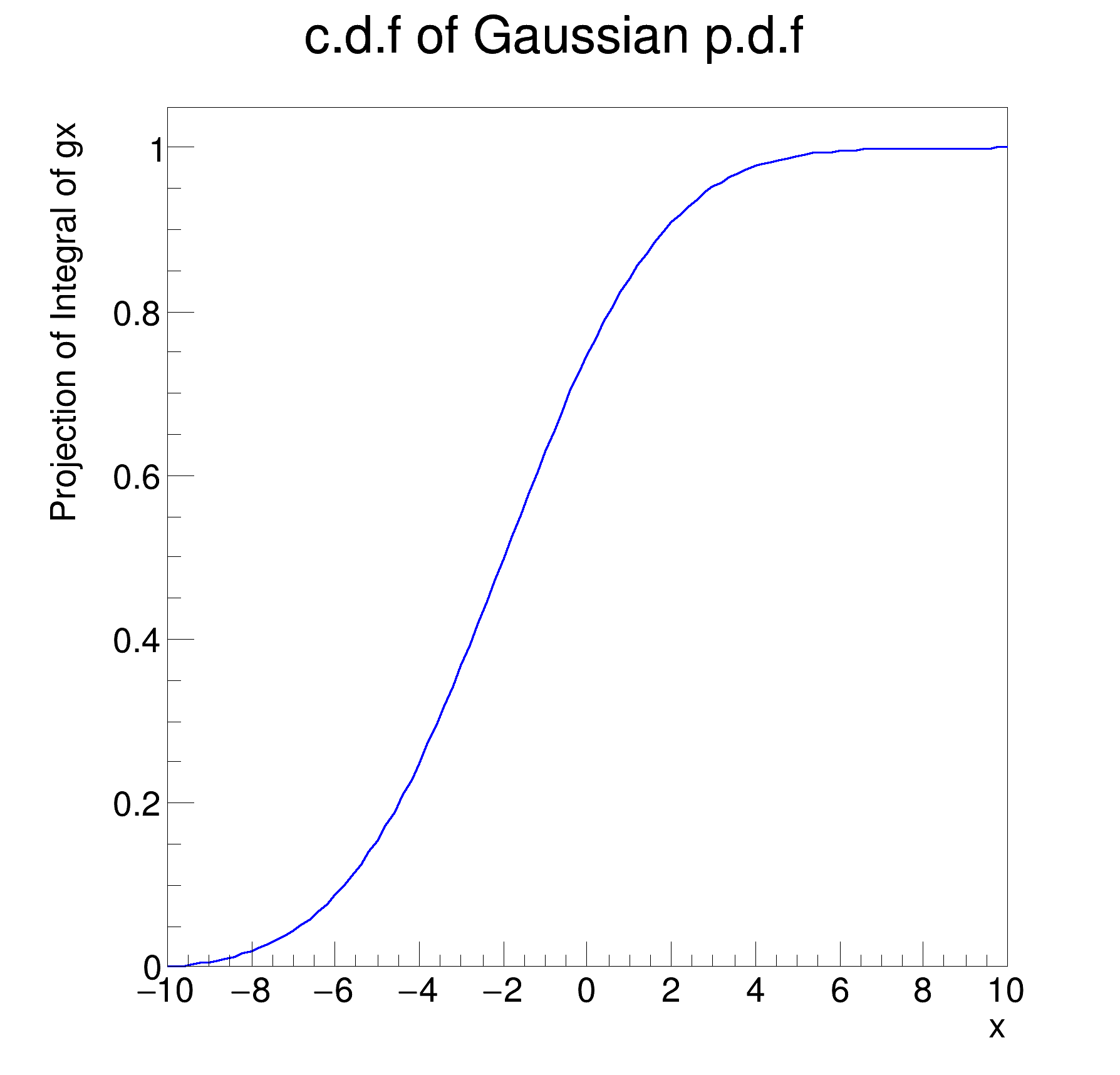ROOT   Reference Guiderf110_normintegration.C File Reference

## Detailed Description

Basic functionality: normalization and integration of p.d.fs, construction of cumulative distribution monodimensional functions␛[1mRooFit v3.60 -- Developed by Wouter Verkerke and David Kirkby␛[0m
Copyright (C) 2000-2013 NIKHEF, University of California & Stanford University
gx = 0.800737
gx_Norm[x] = 0.106896
gx_Int[x] = 7.49084
[#1] INFO:Eval -- RooRealVar::setRange(x) new range named 'signal' created with bounds [-5,5]
gx_Int[x|signal]_Norm[x] = 0.834753
#include "RooRealVar.h"
#include "RooGaussian.h"
#include "RooConstVar.h"
#include "RooAbsReal.h"
#include "RooPlot.h"
#include "TCanvas.h"
#include "TAxis.h"
using namespace RooFit;
{
// S e t u p m o d e l
// ---------------------
// Create observables x,y
RooRealVar x("x", "x", -10, 10);
// Create p.d.f. gaussx(x,-2,3)
RooGaussian gx("gx", "gx", x, RooConst(-2), RooConst(3));
// R e t r i e v e r a w & n o r m a l i z e d v a l u e s o f R o o F i t p . d . f . s
// --------------------------------------------------------------------------------------------------
// Return 'raw' unnormalized value of gx
cout << "gx = " << gx.getVal() << endl;
// Return value of gx normalized over x in range [-10,10]
RooArgSet nset(x);
cout << "gx_Norm[x] = " << gx.getVal(&nset) << endl;
// Create object representing integral over gx
// which is used to calculate gx_Norm[x] == gx / gx_Int[x]
cout << "gx_Int[x] = " << igx->getVal() << endl;
// I n t e g r a t e n o r m a l i z e d p d f o v e r s u b r a n g e
// ----------------------------------------------------------------------------
// Define a range named "signal" in x from -5,5
x.setRange("signal", -5, 5);
// Create an integral of gx_Norm[x] over x in range "signal"
// This is the fraction of of p.d.f. gx_Norm[x] which is in the
// range named "signal"
RooAbsReal *igx_sig = gx.createIntegral(x, NormSet(x), Range("signal"));
cout << "gx_Int[x|signal]_Norm[x] = " << igx_sig->getVal() << endl;
// C o n s t r u c t c u m u l a t i v e d i s t r i b u t i o n f u n c t i o n f r o m p d f
// -----------------------------------------------------------------------------------------------------
// Create the cumulative distribution function of gx
// i.e. calculate Int[-10,x] gx(x') dx'
RooAbsReal *gx_cdf = gx.createCdf(x);
// Plot cdf of gx versus x
RooPlot *frame = x.frame(Title("c.d.f of Gaussian p.d.f"));
gx_cdf->plotOn(frame);
// Draw plot on canvas
new TCanvas("rf110_normintegration", "rf110_normintegration", 600, 600);
frame->GetYaxis()->SetTitleOffset(1.6);
frame->Draw();
}
RooAbsReal is the common abstract base class for objects that represent a real value and implements f...
Definition: RooAbsReal.h:60
virtual RooPlot * plotOn(RooPlot *frame, const RooCmdArg &arg1=RooCmdArg(), const RooCmdArg &arg2=RooCmdArg(), const RooCmdArg &arg3=RooCmdArg(), const RooCmdArg &arg4=RooCmdArg(), const RooCmdArg &arg5=RooCmdArg(), const RooCmdArg &arg6=RooCmdArg(), const RooCmdArg &arg7=RooCmdArg(), const RooCmdArg &arg8=RooCmdArg(), const RooCmdArg &arg9=RooCmdArg(), const RooCmdArg &arg10=RooCmdArg()) const
Plot (project) PDF on specified frame.
Double_t getVal(const RooArgSet *normalisationSet=nullptr) const
Evaluate object.
Definition: RooAbsReal.h:90
RooAbsReal * createIntegral(const RooArgSet &iset, const RooCmdArg &arg1, const RooCmdArg &arg2=RooCmdArg::none(), const RooCmdArg &arg3=RooCmdArg::none(), const RooCmdArg &arg4=RooCmdArg::none(), const RooCmdArg &arg5=RooCmdArg::none(), const RooCmdArg &arg6=RooCmdArg::none(), const RooCmdArg &arg7=RooCmdArg::none(), const RooCmdArg &arg8=RooCmdArg::none()) const
Create an object that represents the integral of the function over one or more observables listed in ...
Definition: RooAbsReal.cxx:560
RooArgSet is a container object that can hold multiple RooAbsArg objects.
Definition: RooArgSet.h:28
Plain Gaussian p.d.f.
Definition: RooGaussian.h:25
A RooPlot is a plot frame and a container for graphics objects within that frame.
Definition: RooPlot.h:44
TAxis * GetYaxis() const
Definition: RooPlot.cxx:1277
virtual void Draw(Option_t *options=0)
Draw this plot and all of the elements it contains.
Definition: RooPlot.cxx:712
RooRealVar represents a variable that can be changed from the outside.
Definition: RooRealVar.h:35
virtual void SetTitleOffset(Float_t offset=1)
Set distance between the axis and the axis title.
Definition: TAttAxis.cxx:294
The Canvas class.
Definition: TCanvas.h:27
RooCmdArg NormSet(const RooArgSet &nset)
RooConstVar & RooConst(Double_t val)
Double_t x[n]
Definition: legend1.C:17
The namespace RooFit contains mostly switches that change the behaviour of functions of PDFs (or othe...
const char * Title
Definition: TXMLSetup.cxx:67
Ta Range(0, 0, 1, 1)
Date
07/2008

Definition in file rf110_normintegration.C.